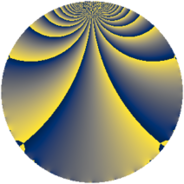# Properties

 Label 760.2.fLevel $760$ Weight $2$ Character orbit 760.f Rep. character $\chi_{760}(381,\cdot)$ Character field $\Q$ Dimension $72$ Newform subspaces $2$ Sturm bound $240$ Trace bound $1$

# Related objects

## Defining parameters

 Level: $$N$$ $$=$$ $$760 = 2^{3} \cdot 5 \cdot 19$$ Weight: $$k$$ $$=$$ $$2$$ Character orbit: $$[\chi]$$ $$=$$ 760.f (of order $$2$$ and degree $$1$$) Character conductor: $$\operatorname{cond}(\chi)$$ $$=$$ $$8$$ Character field: $$\Q$$ Newform subspaces: $$2$$ Sturm bound: $$240$$ Trace bound: $$1$$ Distinguishing $$T_p$$: $$3$$

## Dimensions

The following table gives the dimensions of various subspaces of $$M_{2}(760, [\chi])$$.

Total New Old
Modular forms 124 72 52
Cusp forms 116 72 44
Eisenstein series 8 0 8

## Trace form

 $$72q + 4q^{2} - 4q^{4} - 12q^{6} + 8q^{7} + 16q^{8} - 72q^{9} + O(q^{10})$$ $$72q + 4q^{2} - 4q^{4} - 12q^{6} + 8q^{7} + 16q^{8} - 72q^{9} + 8q^{12} + 8q^{14} - 12q^{16} - 28q^{18} - 8q^{20} - 8q^{22} + 8q^{23} - 4q^{24} - 72q^{25} + 12q^{26} - 4q^{28} + 8q^{30} - 16q^{32} + 32q^{34} + 16q^{36} - 48q^{39} + 4q^{42} + 24q^{46} - 40q^{47} + 12q^{48} + 72q^{49} - 4q^{50} + 12q^{52} + 36q^{54} + 16q^{55} + 32q^{56} - 52q^{58} - 28q^{60} - 24q^{62} + 40q^{63} - 4q^{64} + 72q^{66} - 44q^{68} - 8q^{70} - 16q^{71} - 4q^{72} + 40q^{74} - 88q^{78} + 80q^{79} - 8q^{80} + 88q^{81} + 24q^{82} - 56q^{84} - 88q^{86} + 96q^{87} + 60q^{88} - 16q^{89} - 12q^{92} - 16q^{95} + 84q^{96} - 4q^{98} + O(q^{100})$$

## Decomposition of $$S_{2}^{\mathrm{new}}(760, [\chi])$$ into newform subspaces

Label Dim. $$A$$ Field CM Traces $q$-expansion
$$a_2$$ $$a_3$$ $$a_5$$ $$a_7$$
760.2.f.a $$28$$ $$6.069$$ None $$2$$ $$0$$ $$0$$ $$4$$
760.2.f.b $$44$$ $$6.069$$ None $$2$$ $$0$$ $$0$$ $$4$$

## Decomposition of $$S_{2}^{\mathrm{old}}(760, [\chi])$$ into lower level spaces

$$S_{2}^{\mathrm{old}}(760, [\chi]) \cong$$ $$S_{2}^{\mathrm{new}}(40, [\chi])$$$$^{\oplus 2}$$$$\oplus$$$$S_{2}^{\mathrm{new}}(152, [\chi])$$$$^{\oplus 2}$$